# - 7th grade (12y) - math problems

#### Number of problems found: 2730

• Unit costLast Friday, the Math Society sold newspapers to raise money. Each pack weighed 65.5 kg and sold for 281.65 pesos. How much was the cost per kilogram?
• Mass fraction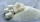2 teaspoons (24 g) of sugar are dissolved in a cup with 500 g of tea. Calculate the weight fraction of sugar in the tea.
• When 5When 5 is subtracted from both my numerator and denominator I become 3/4. What fraction i am?
• A plumber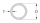A plumber has the following lengths of PVC: 3.3 ft, 9.77 ft, 8.41 ft, 9.61 ft, 8 ft. What is his average length of PVC
• AlexandraAlexandra made a rectangular quilt the measured 3 1/4. 2 3/4 feet in width. What is the area of the quilt in square feet? Write an equation to solve.
• Frame image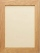Peter wants to put a picture in a frame. The picture is 7 3/5 cm wide. To fit in the picture frame cannot be more than 7 3/10 cm wide. How much should the image be trimmed?
• A rectangle 7A rectangle that has been split into 24 parts, set in 4 rows of 6 parts each. The parts in the top 3 rows have been shaded blue. The parts in the left 5 columns have been shaded purple. The first 5 parts in the top 3 rows are striped to show they are shad
• Three integersThree consecutive integers add up to 51. what are these integers?
• Original numberJoe divided a number by 2 1/4 to get 2/3. What was the original number?
• ParallogramParallogram base is 24 cm and high 10 cm. How many such tile are required to cover a floor of area 1080m2?
• The product 2The product of two functions is 10. If one of them is 2 1/3, find the other one.
• Multiplicative inverse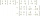Explain why the reciprocal of - 1 1/17 is the multiplicative inverse of - 17/18.
• Fraction equationEx over four is equal to two and one-fifth times ex plus three over ten. What is ex>
• Simplify expression with mixedWhen (7¹/3 + 2¾)÷(2 + 2¼ × 1¹/3) is simplified the result is?
• Frac equation v23.80 is two fifths of a quarter sum of money. How much money is there?
• Two fraction equation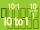Find d value of q in the equation 30/16=q/48.
• Quotient and divisionWhat is the quotient of 2/9 and 1/8?
• A class IV.CIn a class 2/5 were boys. 30 were girls. How many more girls than boys are there?
• Calculate 20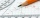Calculate the sum of 1/5 of a right angle and 3/4 of a right angle and 3/4 of a straight angle
• Licorice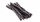A piece of licorice is 2/3 yard long.  If it is cut into 10 equal pieces, how long will each piece of licorice be?

Do you have an exciting math question or word problem that you can't solve? Ask a question or post a math problem, and we can try to solve it.

We will send a solution to your e-mail address. Solved examples are also published here. Please enter the e-mail correctly and check whether you don't have a full mailbox.

Please do not submit problems from current active competitions such as Mathematical Olympiad, correspondence seminars etc...